##### B.S. in Mathematics

Checksheet & Suggested Course Sequence for students who enrolled at Kutztown in Fall 2018 or later
Checksheet & Suggested Course Sequence for students who enrolled at Kutztown before Fall 2018

The Department of Mathematics offers a broad range of courses, which provide a solid preparation for students with a wide spectrum of needs: from those who wish to pursue further studies in mathematics or related subjects, to those who wish to find highly-rewarding jobs in the industry.

Our program provides a solid array of courses from applied mathematical sciences and pure theoretical mathematics.  A B.S. in mathematics comes with an applied or pure specialization, with a mixture of essential courses from both branches in the core component of the major.  Below, we provide a small gallery of several courses from each specialization to give an idea of the type of mathematics that students encounter at KU.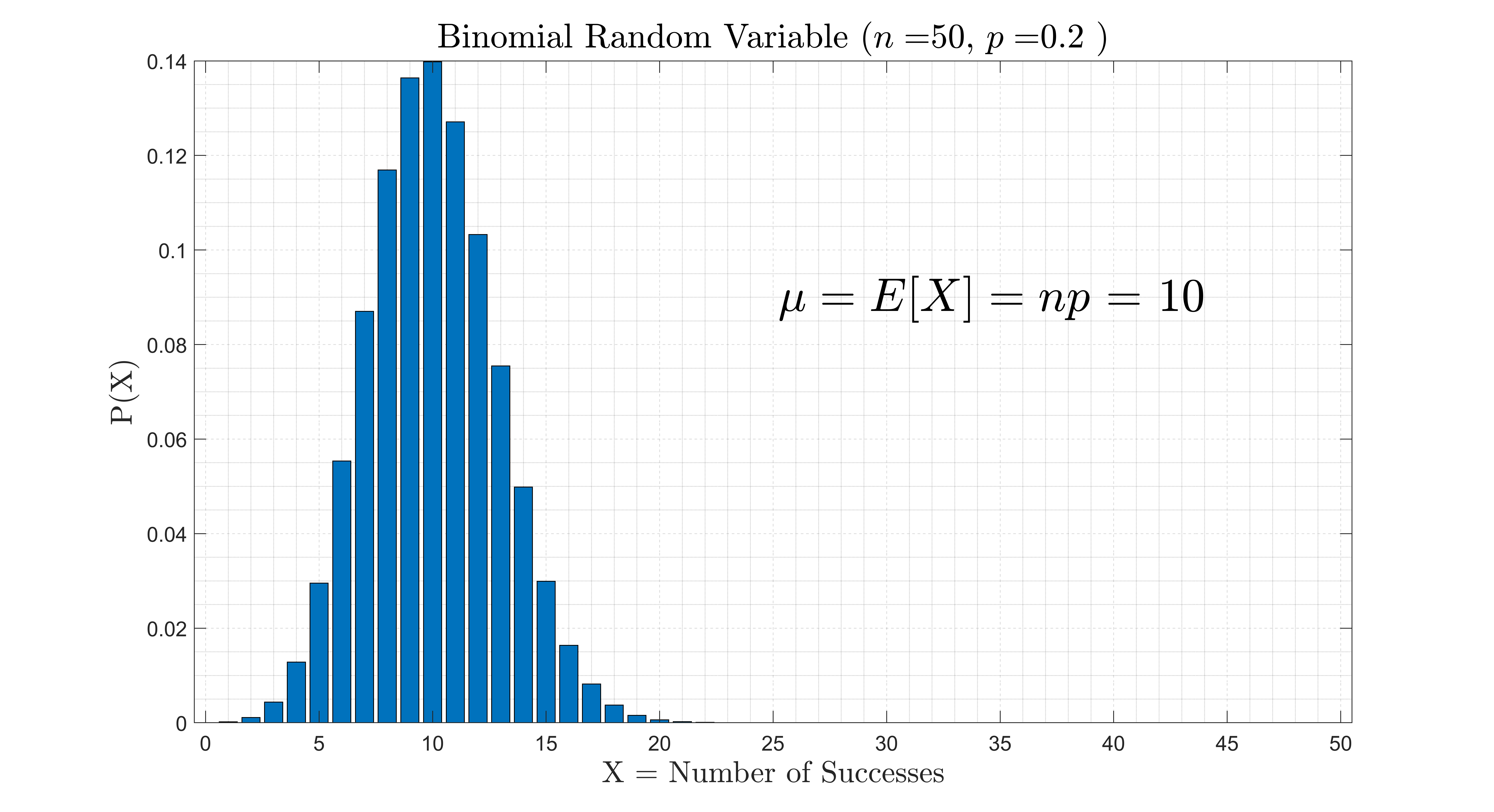## MAT 301/302 -- Probability and Statistics

Probability and statistics are essential for the applied math specialization. The fundamentals covered in this class prepare the student for a wide array of mathematical applications. The figure depicts the binomial distribution, which is one of the first discrete probability distributions encountered. This distribution has wide applications from shooting free throws to determining the efficacy of a drug.## MAT 311/312 -- Abstract Algebra

Abstract Algebra is a core course for Mathematics and Secondary Education Mathematics majors. Students study familiar concepts about sets, operations, and functions in a rigorous way, and apply them to unfamiliar abstract settings, which often leads to interesting and surprising consequences. This figure depicts a subgroup lattice for the group Z_980. Lattices such as this help us to visualize the structure of objects and investigate how objects may be similar to, or different from, one another.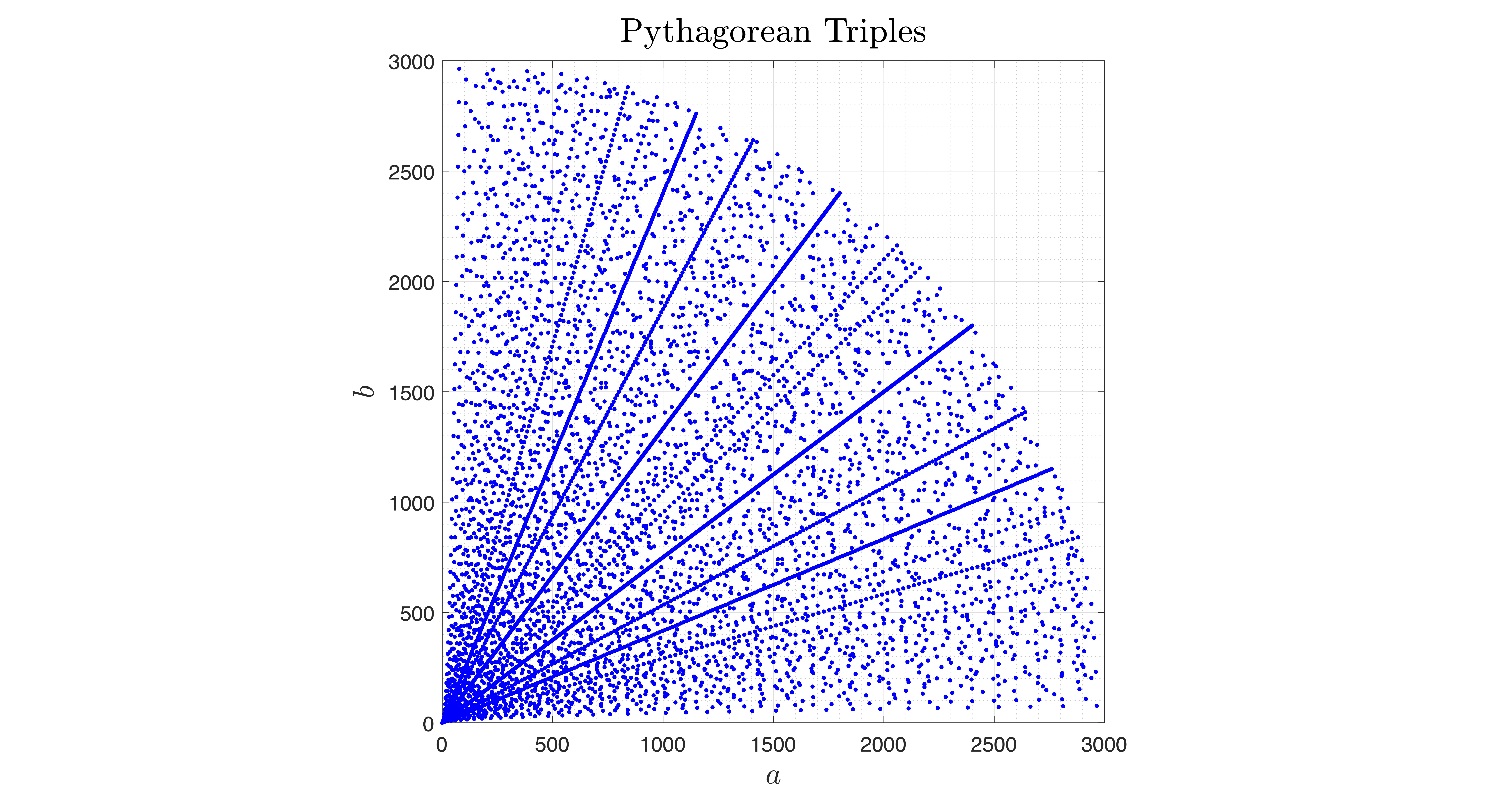## MAT 330 -- Theory of Numbers

The Theory of Numbers is a classical study of the integers and integer-valued functions. This course is a higher-level introductory course that is a staple of the pure mathematics specialization. The course typically starts with the Fundamental Theorem of Arithmetic and works its way to higher-level ideas such as Diophantine equations and cryptography. The figure depicts the set of ordered pairs (a,b) in the first quadrant such that a, b, and c are Pythagorean triples that satisfy a^2 + b^2 = c^2. For example, (3,4,5) is a Pythagorean triple and so the point (3,4) is plotted with a blue dot.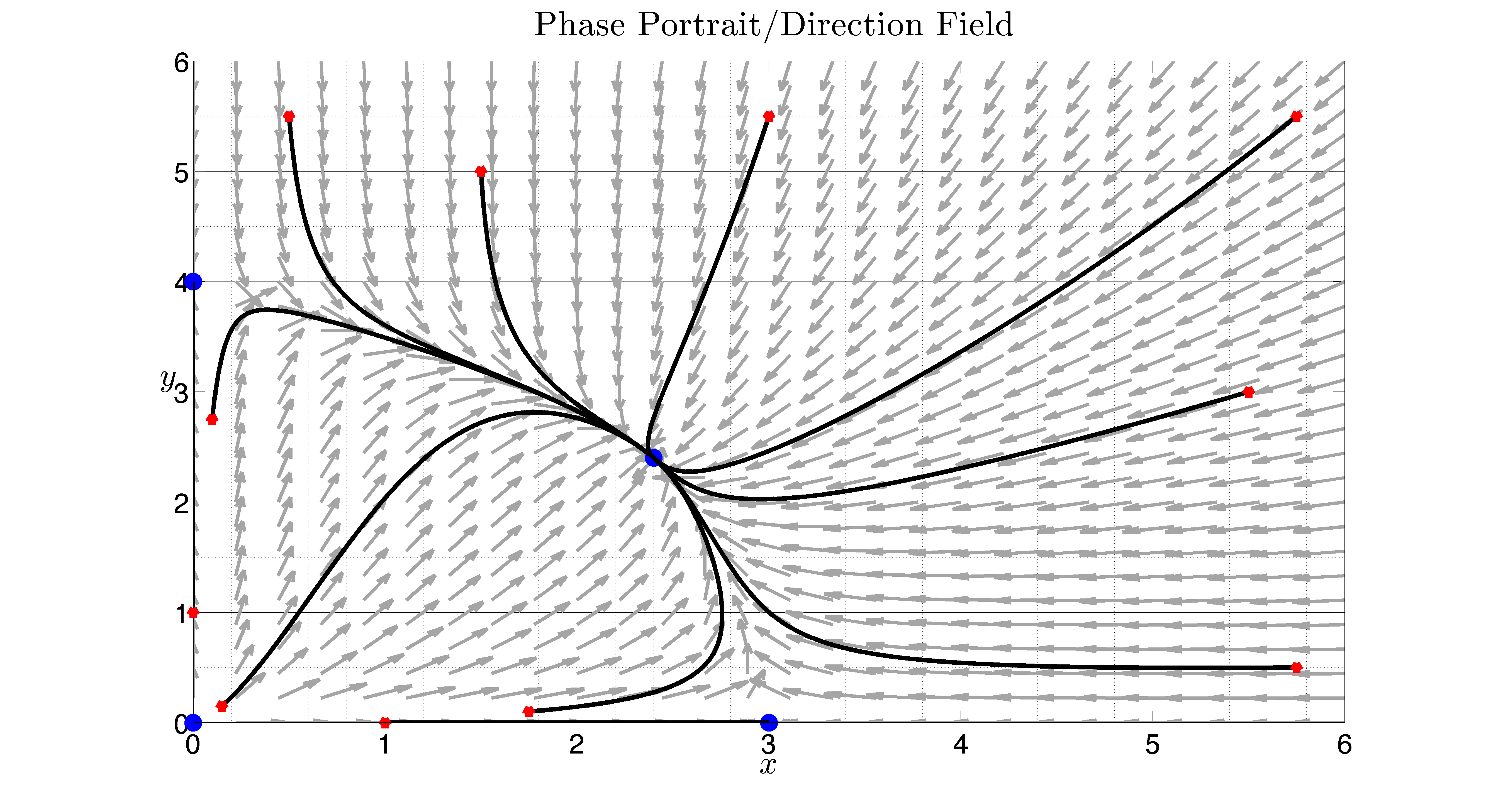## MAT 340 -- Differential Equations

This course is a core requirement for the applied specialization. Differential equations are essentially the universal language for describing how quantities continuously change over time. Other disciplines make use of the predictive power of differential equations, including biology, chemistry, physics, engineering, and even the social sciences. The figure depicts the phase portrait of a competing species model with several trajectories. In short, if two quantities (x and y) start at any of the red dots, they will both progress to a common non-zero equilibrium at the center blue dot. In other words, they'll coexist.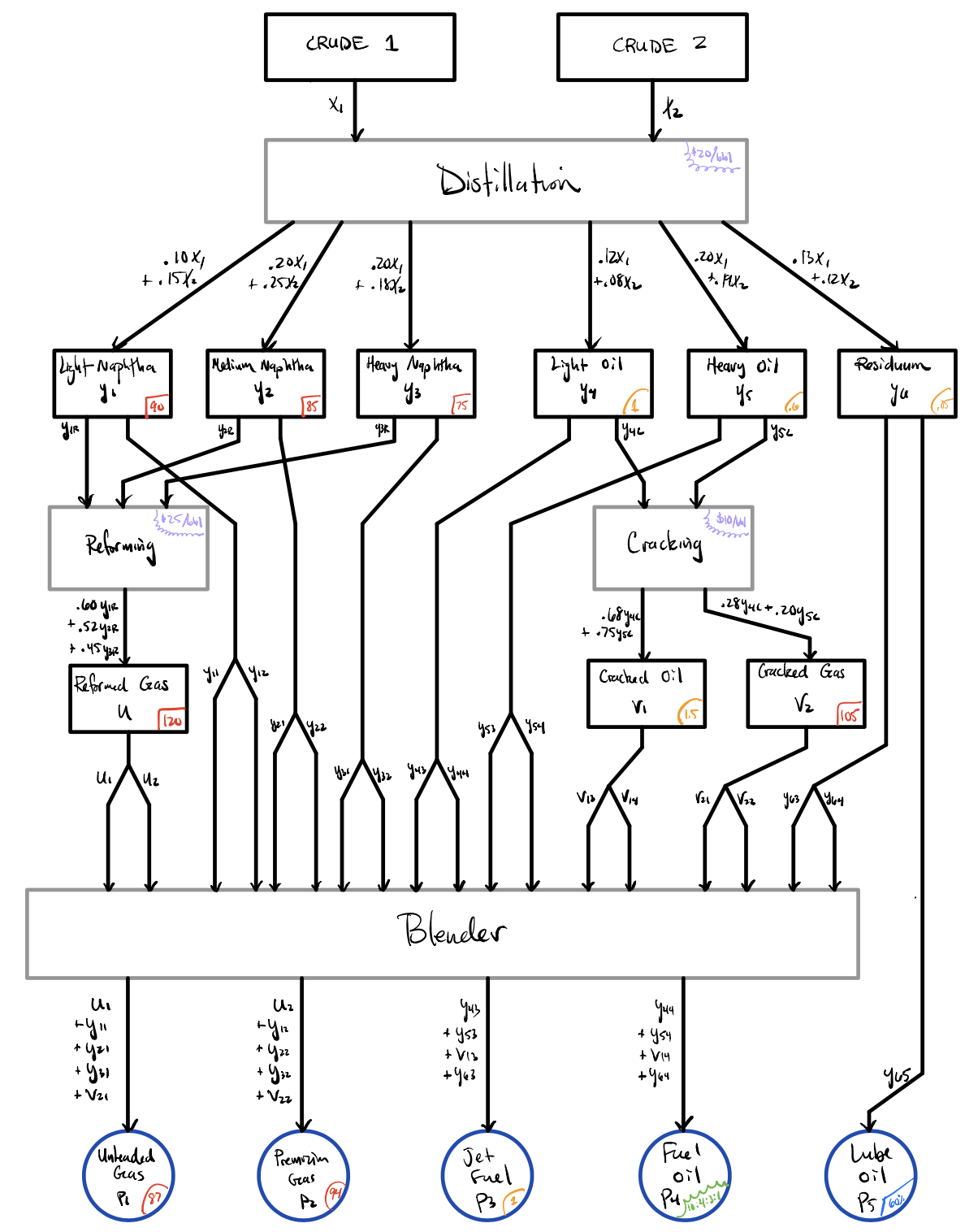## MAT 361/362 -- Operations Research

This course sequence is a cornerstone of the applied math specialization. Operations research is the study of optimization through various programming methods. For instance, in this course, the student will learn to maximize revenue under limited resource availability or minimize calorie intake given a specific set of food requirements. This figure depicts a schematic of a classic blending problem that takes in two different types of crude oils and outputs five products. The mathematical model implemented will maximize the profits by determining the optimal production schedule based on several distilling and cracking processes.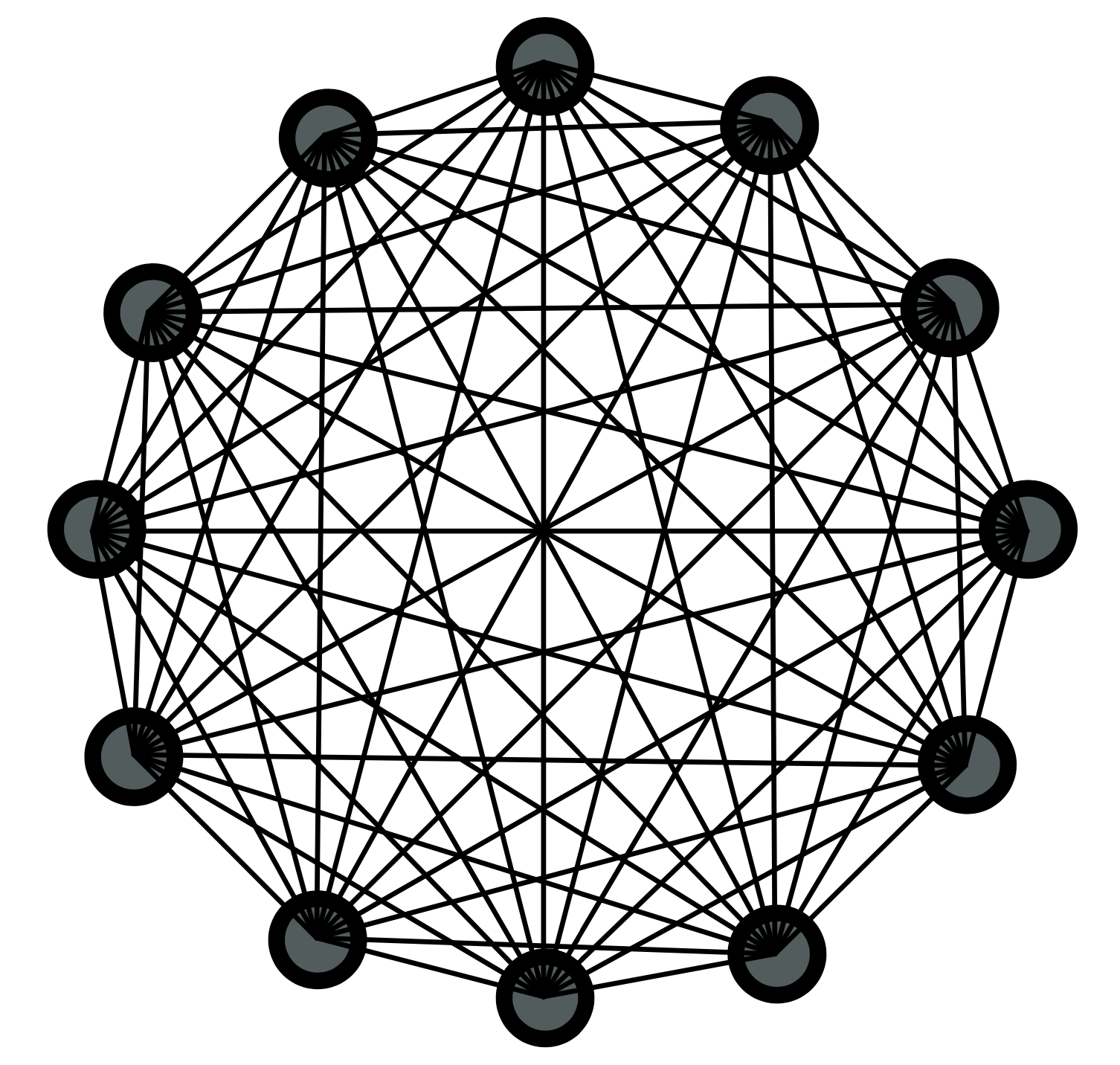## MAT 369 -- Introduction to Graph Theory

Graph Theory is the popular study of mathematical networks. A graph or network is a structure consisting of a set of nodes or vertices connected by edges or arcs. The versatility of the graph structure is apparent in many other disciplines including computer science where graphs represent data structures and in sociology where networks may represent the strength of human relationships. This course is an introduction into the mathematical analysis of different types of graphs and subgraphs, including ideas such as connectivity, Euler tours, and Hamiltonian cycles. The figure depicts the complete graph of 12 vertices and 66 edges. A complete graph is a network in which every pair of vertices is connected by a unique edge.##### Minor in Mathematics

Checksheet

Employers seek employees with the problem-solving skills that are found in mathematics courses.  Students who wish to be competitive in their various careers may want to strengthen their mathematical background by pursuing a minor in mathematics.

##### Minor in Actuarial Science

Checksheet

Actuaries are business professionals who deal with the financial impact of risk and uncertainty.  They analyze, manage, and measure the financial implications of future risk, as well as develop and validate models and communicate results to guide decision-making.  This program helps students develop the fundamental knowledge and skills vital to meeting the demands of an actuarial career, as well as prepares students for two of the required national actuarial exams.